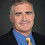## Friday, December 02, 2005

### Complexity in the Mid-80's

Luca asked about the topics in the complexity courses I took in 1985 and 1986. I dug up my old course notes and wrote down what was covered. Some more in the intersection than I remembered.

Cornell University CS 682–Theory of Computation
Spring 1985
Juris Hartmanis

Recursion Theorem, Rice's Theorem, Myhill's theorem that all r.e.-complete sets are recursively isomorphic, Kolmogorov complexity, Relativization, arithmetic hierarchy leading to the polynomial-time hierarchy, Ladner's theorem, oracles for P=NP and P≠NP and some other hypotheses, Blum Speed-Up Theorem, Cook's theorem, isomorphism conjecture for NP-complete sets, Mahaney's theorem (Sparse NP-complete sets imply P=NP), Blum's axioms, probabilistic classes, random oracle hypothesis, E=NE iff no sparse sets in NP-P, Karp-Lipton, Union Theorem, Elementary and Primitive recursive functions.

Much of this course revolved around the isomorphism conjecture that Hartmanis felt at the time could lead to a proof that P ≠ NP.

On 2/18/85 Hartmanis stated that whether there is an oracle where PH is infinite is an open question and on 3/13/85 Hartmanis announced that Yao found such an oracle but no mention of how Yao's proof worked, i.e., no circuit complexity.

UC Berkeley CS 278–Machine-Based Complexity
Spring 1986
Michael Sipser

Turing machines, Cook's Theorem, Savitch's theorem, Path is NL-complete, alternation and PSPACE-completeness, Circuit valuation is P-complete and P/poly, Oracles and Random Oracles, Probabilistic classes, Undirected path in RL (now known to be in L), Graph Isomorphism in co-AM, Kolmogorov complexity, using Kolmogorov complexity to show BPP in the hierarchy, Karp-Lipton, Mahaney's theorem, Parallel Computation (NC and AC), CFL in NC2, Parity not in AC0 (Furst-Saxe-Sipser, 4 lectures with mention of Yao and Hastad's improvements), Connections to oracles and bounds on depth-k circuits, Razborov's proof that CLIQUE does not have poly-size monotone circuits (6 lectures), Barrington's Theorem (bounded-width branching programs=NC1).

Sipser ended the course talking about his approach to showing NP ≠ co-NP by trying to find a finite analogue of a combinatorial proof that the analytic sets are not closed under complement.

Many of the theorems in Sipser's course were proven after the start of Hartmanis' course such as graph isomorphism in co-AM and Razborov's theorem.

1.UC Berkeley? I thought you attended

2.I started graduate school at Berkeley but when Sipser, my advisor, moved back to MIT in the summer of '86, I followed.

3."Much of this course revolved around the isomorphism conjecture that Hartmanis felt at the time could lead to a proof that P ? NP."

"Sipser ended the course talking about his approach to showing NP ? co-NP"

Things surely have changed in 20 years.

4.More accurately in this regard, very little has changed in the last 20 years.

5.Today it seems that at best such a course might end with an approach to separate #P from ACC^0.

6.The main thing is that it would have to include Razborov-Rudich's natural proofs paper.